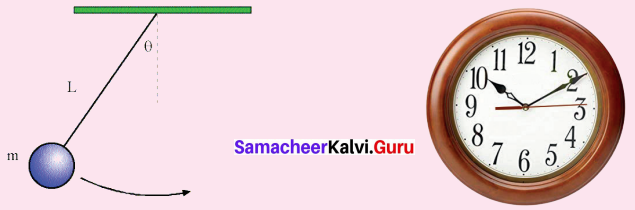Students can Download Maths Chapter 2 Measurements Intext Questions and Answers, Notes Pdf, Samacheer Kalvi 7th Maths Book Solutions Guide Pdf helps you to revise the complete Tamilnadu State Board New Syllabus and score more marks in your examinations.

## Tamilnadu Samacheer Kalvi 7th Maths Solutions Term 2 Chapter 2 Measurements Intext Questions

Exercise 2.1

Try These (Text book Page No. 23)

Question 1.
A few real life examples of circular shapes are given below.Can you give three more examples.
Solution:

1. One and Two rupee coins
2. Bangles
3. Mouth of Bottle

Question 2.
Find the diameter of your bicycle wheel?
Solution:
Diameter of my bicycle wheel is 700 mm

Question 3.
If the diameter of the circle is 14cm, what will be it’s radius?
Solution:
diameter d = 14 cm
radius = $$\frac { d }{ 2 }$$ = $$\frac { 14 }{ 2 }$$ = 7cm

Question 4.
If the radius of a bangle is 2 inches then find the diameter.
Solution:
Given radius of the bangle = 2 inches
Diameter = 2 × radius = 2 × 2 = 4 inchesExercise 2.2

Try These (Text book Page No. 33)

Question 1.
Draw circles of different radii on a graph paper. Find the area by counting the number of squares covered by the circle. Also find the area by using the formula.
(i) Find the area of the circle, if the radius is 4.2 cm.
(ii) Find the area of the circle if the diameter is 28 cm.
Solution:
(i) Radius of the circle r = 4.2 cm
Area of the circle A = π r2 sq.units
= $$\frac { 22 }{ 7 }$$ × 4.2 × 4.2 cm2 = 5.44 cm2

(ii) Diameter of the circle d = 28 cm
radius r = $$\frac { d }{ 2 }$$ = $$\frac { 28 }{ 2 }$$ = 14 cm
Area of the circle A = π r2 sq.units
= $$\frac { 22 }{ 7 }$$ × 14 × 14 cm2 = 616 cm2Exercise 2.3

Try These (Text book Page No. 35)

Question 1.
If the outer radius and inner radius of the circles are respectively 9 cm and 6 cm, find the width of the circular pathway.
Solution:
Radius of the outer circle R = 9 cm
Radius of the inner circle r = 6 cm
Width of the circular pathway = Radius of the outer circle
– Radius of the inner circle
= (9 – 6) cm = 3 cm
Width of the circular pathway = 3 cmQuestion 2.
If the area of the circular pathway is 352 sq.cm and the outer radius is 16 cm, find the inner radius.
Solution:
Given outer radius R = 16 cm
Area of the circular pathway = πR2 = πr2
Area of the circular pathway = 352 sq. cm
πR2 – πr2 = 352 cm2
π(R2 – r2) = 352
162 – r2 = $$\frac{352 \times 7}{22}$$
162 – r2 = 16 × 7
162 – r2 = 112
162 – 112 = r2
r2 = 256 – 112
r2 = 144
r = 12 cm
Inner radius r = 12 cm

Question 3.
If the area of the inner rectangular region is 15 sq.cm and the area covered by the outer rectangular region is 48 sq.cm, find the area of the rectangular pathway. Area of the outer rectangle Area of the inner rectangle Area of the rectangular pathway
Solution:
Area of the outer rectangle = 48 sq.cm
Area of the inner rectangle = 15 sq.cm
Area of the rectangular pathway = Area of the outer rectangle
– Area of the inner rectangle
= 48 – 15 = 33 cm2